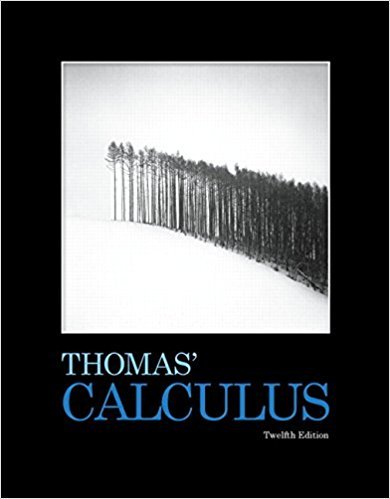×
Get Full Access to Thomas' Calculus - 12 Edition - Chapter 4 - Problem 4.37
Get Full Access to Thomas' Calculus - 12 Edition - Chapter 4 - Problem 4.37

×

# Each of Exercises 33-38 gives the first | Ch 4 - 37ISBN: 9780321587992 158

## Solution for problem 4.37 Chapter 4

Thomas' Calculus | 12th Edition

• Textbook Solutions
• 2901 Step-by-step solutions solved by professors and subject experts
• Get 24/7 help from StudySoup virtual teaching assistantsThomas' Calculus | 12th Edition

4 5 1 308 Reviews
24
5
Problem 4.37

Each of Exercises 33-38 gives the first derivative of a function y ~ f(x). (8) At what points, if any, does the graph of f have a local maximum, local mininmm, or inflection point? (b) Sketch the general abape of the graph y' = x4 - 2,x2

Step-by-Step Solution:
Step 1 of 3

Calculus notes for week of 9/19/16 3.6 Derivatives as Rates of Change Velocity is measured as: V ave(t+∆t) or s(b) – s(a) ∆t b – a (Change in position over change in time.) S’’(t) = V’(t) = A(t) (From left to right: S=Position, V= Velocity, and A=Acceleration) Average and Marginal Cost Suppose C(x) gives the total cost to produce x units of a good cost. Sometimes, C(x) = FC + VC * x FC = Fixed cost which does not change with units produced. VC = Variable cost which is the cost to produce each unit. C(x) = Average cost. C’(x) = Marginal cost, which is approximately the extra cost to produce one more unit beyond x units. C’(x) = lim C(x+∆x) – C(x) ∆x>0 ∆x 3.7 Chain Rule How do we differentiate a composi

Step 2 of 3

Step 3 of 3

##### ISBN: 9780321587992

Unlock Textbook Solution